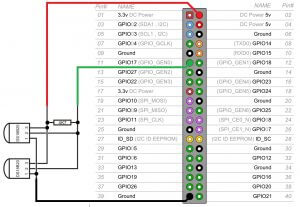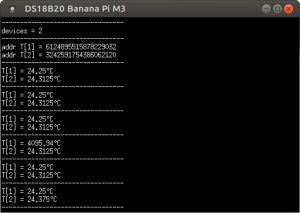# Connecting DS18B20 Temperature Sensor to Orange Pi, Banana Pi, Raspberry Pi

The DS18B20 is perhaps one of the most famous and affordable temperature sensors available. Mostly microcontrollers are used to read data from DS18B20, for example: ATmega8 , ATtiny2313 , Arduino, etc. With the advent of single-board mini-computers, it became interesting how the connected DS18B20 temperature sensor would work to Orange Pi, Banana Pi or Raspberry Pi - the most popular mini computers.
To work with GPIOs on Orange Pi and Banana Pi, you need to install WiringOP and BPI-WiringPi, respectively, and IDE Code :: Blocks
was selected when creating the article, since I have it on all the time. But this sample program will work when the DS18B20 is connected to an Orange Pi or Raspberry Pi.

## OneWire library

### OneWire.h

`#ifndef ONEWIRE_H#define ONEWIRE_H#define CMD_CONVERTTEMP    0x44#define CMD_RSCRATCHPAD    0xbe#define CMD_WSCRATCHPAD    0x4e#define CMD_CPYSCRATCHPAD  0x48#define CMD_RECEEPROM      0xb8#define CMD_RPWRSUPPLY     0xb4#define CMD_SEARCHROM      0xf0#define CMD_READROM        0x33#define CMD_MATCHROM       0x55#define CMD_SKIPROM        0xcc#define CMD_ALARMSEARCH    0xec#include <stdint.h>class OneWire {private:  int pin;  uint64_t searchNextAddress(uint64_t, int&);public:  OneWire(int);  virtual ~OneWire();  int reset(void);  int crcCheck(uint64_t, uint8_t);  uint8_t crc8(uint8_t*, uint8_t);  void oneWireInit();  void writeBit(uint8_t);  void writeByte(uint8_t);  void setDevice(uint64_t);  void searchRom(uint64_t*, int&);  void skipRom(void);  uint8_t readByte(void);  uint8_t readBit(void);  uint64_t readRoom(void);};#endif // ONEWIRE_H`

### OneWire.cpp

`#include "OneWire.h"#include <wiringPi.h>#include <stdexcept>#include <iostream>OneWire::OneWire(int _pin) :    pin(_pin) {}OneWire::~OneWire() {}void OneWire::oneWireInit() {  if (wiringPiSetup() == -1) {    throw std::logic_error("WiringPi Setup error");  }  pinMode(pin, INPUT);}/* * reset */int OneWire::reset() {  int response;  pinMode(pin, OUTPUT);  digitalWrite(pin, LOW);  delayMicroseconds(480);  // When the ONE WIRE device detects a positive edge, it waits for 15us to 60us   pinMode (pin, INPUT);  delayMicroseconds ( 60 );  // and then transmits a presence pulse, moving the bus to logic "0" for a duration from 60us to 240us.   response = digitalRead (pin);  delayMicroseconds ( 410 );  // if 0, then there is a response from the sensor, if 1 - no   return response;}/* * send one bit */void OneWire::writeBit(uint8_t bit) {  if (bit & 1) {    // логический «0» на 10us    pinMode(pin, OUTPUT);    digitalWrite(pin, LOW);    delayMicroseconds(10);    pinMode(pin, INPUT);    delayMicroseconds(55);  } else {    // логический «0» на 65us    pinMode(pin, OUTPUT);    digitalWrite(pin, LOW);    delayMicroseconds(65);    pinMode(pin, INPUT);    delayMicroseconds(5);  }}/* * send one byte */void OneWire::writeByte(uint8_t byte) {  uint8_t i = 8;  while (i--) {    writeBit(byte & 1);    byte >>= 1;  }}/* * get one byte */uint8_t OneWire::readByte() {  uint8_t i = 8, byte = 0;  while (i--) {    byte >>= 1;    byte |= (readBit() << 7);  }  return byte;}/* * get one bit */uint8_t OneWire::readBit(void) {  uint8_t bit = 0;  // логический «0» на 3us  pinMode(pin, OUTPUT);  digitalWrite(pin, LOW);  delayMicroseconds(3);  // release the line and wait 10us   pinMode (pin, INPUT);  delayMicroseconds ( 10 );  // read the value   bit = digitalRead (pin);  // wait for 45us and return   delayMicroseconds ( 45 );  return bit;}/* * read slave ROM (64 bit code) */uint64_t OneWire::readRoom(void) {  uint64_t oneWireDevice;  if (reset() == 0) {    writeByte (CMD_READROM);    //  код семейства    oneWireDevice = readByte();    // серийный номер    oneWireDevice |= (uint16_t) readByte() << 8 | (uint32_t) readByte() << 16 | (uint32_t) readByte() << 24 | (uint64_t) readByte() << 32 | (uint64_t) readByte() << 40        | (uint64_t) readByte() << 48;    // CRC    oneWireDevice |= (uint64_t) readByte() << 56;  } else {    return 1;  }  return oneWireDevice;}/* * ROM match command followed by a sequence * 64 bit ROM code allows bus control device * access a specific slave on the bus. */void OneWire::setDevice(uint64_t rom) {  uint8_t i = 64;  reset();  writeByte (CMD_MATCHROM);  while (i--) {    writeBit(rom & 1);    rom >>= 1;  }}/* * CRC check, returns "0" if there are no errors * and not "0" if there are errors */int OneWire::crcCheck(uint64_t data8x8bit, uint8_t len) {  uint8_t dat, crc = 0, fb, stByte = 0;  do {    dat = ( uint8_t ) (data8x8bit >> (stByte * 8 ));    // counter of bits in byte     for ( int i = 0 ; i < 8 ; i ++) {      fb = crc ^ that;      fb &= 1;      crc >>= 1;      dat >>= 1;      if (fb == 1) {        crc ^ = 0x8c ; // polynomial      }    }    stByte ++;  } while (stByte <len);   // count of bytes in the array   return crc;}uint8_t OneWire::crc8(uint8_t addr[], uint8_t len) {  uint8_t crc = 0;  while (len--) {    uint8_t inbyte = *addr++;    for (uint8_t i = 8; i; i--) {      uint8_t mix = (crc ^ inbyte) & 0x01;      crc >>= 1;      if (mix) {        crc ^= 0x8c;      }      inbyte >>= 1;    }  }  return crc;}/* * device search */void OneWire::searchRom(uint64_t * roms, int & n) {  uint64_t lastAddress = 0;  int lastDiscrepancy = 0;  int err = 0;  int i = 0;  do {    do {      try {        lastAddress = searchNextAddress (lastAddress, lastDiscrepancy);        int crc = crcCheck (lastAddress, 8 );        if (crc == 0 ) {          roms [i ++] = lastAddress;          err = 0;        } else {          err++;        }      } catch (std::exception & e) {        std::cout << e.what() << std::endl;        err++;        if (err > 3) {          throw e;        }      }    } while (err != 0);  } while (lastDiscrepancy != 0 && i < n);  n = i;}/* * search for next connected device */uint64_t OneWire::searchNextAddress(uint64_t lastAddress, int & lastDiscrepancy) {  uint64_t newAddress = 0;  int searchDirection = 0;  int idBitNumber = 1;  int lastZero = 0;  reset();  writeByte (CMD_SEARCHROM);  while (idBitNumber < 65) {    int idBit = readBit();    int cmpIdBit = readBit();    // id_bit = cmp_id_bit = 1    if (idBit == 1 && cmpIdBit == 1) {      throw std::logic_error("error: id_bit = cmp_id_bit = 1");    } else if (idBit == 0 && cmpIdBit == 0) {      // id_bit = cmp_id_bit = 0      if (idBitNumber == lastDiscrepancy) {        searchDirection = 1;      } else if (idBitNumber > lastDiscrepancy) {        searchDirection = 0;      } else {        if ((uint8_t)(lastAddress >> (idBitNumber - 1)) & 1) {          searchDirection = 1;        } else {          searchDirection = 0;        }      }      if (searchDirection == 0) {        lastZero = idBitNumber;      }    } else {      // id_bit != cmp_id_bit      searchDirection = idBit;    }    newAddress |= ((uint64_t) searchDirection) << (idBitNumber - 1);    writeBit(searchDirection);    idBitNumber++;  }  lastDiscrepancy = lastZero;  return newAddress;}/* * skip ROM * / void  OneWire :: skipRom ()  {   reset ();  writeByte (CMD_SKIPROM);}`

## Connecting multiple DS18B20s to Orange Pi on one busWhen connecting multiple DS18B20 sensors to an Orange Pi, Banana Pi, or Raspberry Pi on the same bus, the master device (computer) must determine the ROM codes of all slaves on the bus. The SEARCH ROM [F0h] - (SEARCH ROM) command allows the control to determine the numbers and types of slave devices. The control learns the ROM codes through a remediation process that requires the Master to perform a ROM Search cycle (that is, a Search ROM command followed by a data exchange). This procedure must be performed as many times as necessary to identify all of the slaves. If there is only one slave on the bus, the simpler READ ROM command is[33h] (Read ROM) The place of the Search ROM process can be used.
After each ROM Search cycle, the bus manager must return to Step 1 (Initialization) in the operating sequence.

### main.cpp

`#include <iostream>#include <wiringPi.h>#include "OneWire.h"using namespace std;double getTemp(OneWire * oneWire, uint64_t ds18b20s) {  uint8_t data;  do {    oneWire->setDevice(ds18b20s);    oneWire->writeByte(CMD_CONVERTTEMP);    delay(750);    oneWire->setDevice(ds18b20s);    oneWire->writeByte(CMD_RSCRATCHPAD);    for (int i = 0; i < 9; i++) {      data[i] = oneWire->readByte();    }  } while (oneWire->crc8(data, 8) != data);  return ((data << 8) + data) * 0.0625;}int main() {  OneWire * ds18b20 = new OneWire(24);  try {    ds18b20->oneWireInit();    double temperature;    int n = 100;    uint64_t roms[n];    ds18b20->searchRom(roms, n);    cout << "---------------------------------" << endl;    cout << "devices = " << n << endl;    cout << "---------------------------------" << endl;    for (int i = 0; i < n; i++) {      cout << "addr T[" << (i + 1) << "] = " << roms[i] << endl;    }    cout << "---------------------------------" << endl;    while (1) {      for (int i = 0; i < n; i++) {        temperature = getTemp(ds18b20, roms[i]);        cout << "T[" << (i + 1) << "] = " << temperature << "°C" << endl;      }      cout << "---------------------------------" << endl;      delay(500);    }  } catch (exception & e) {    cout << e.what() << endl;  }}// site: http://micro-pi.ru`

`double getTemp(OneWire * oneWire, uint64_t ds18b20s)` - returns temperature data in degrees Celsius.

### Result